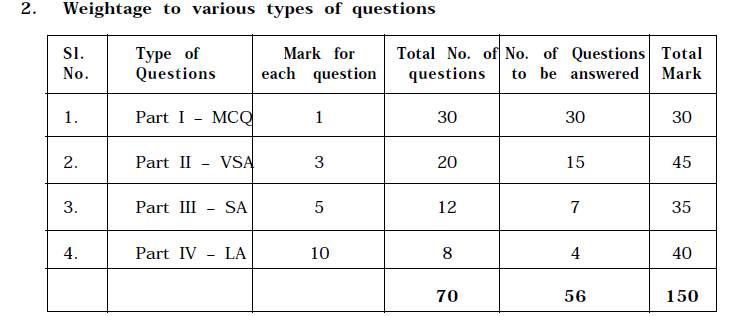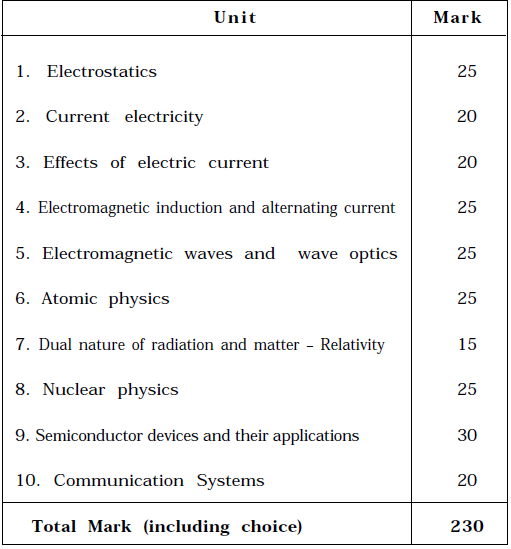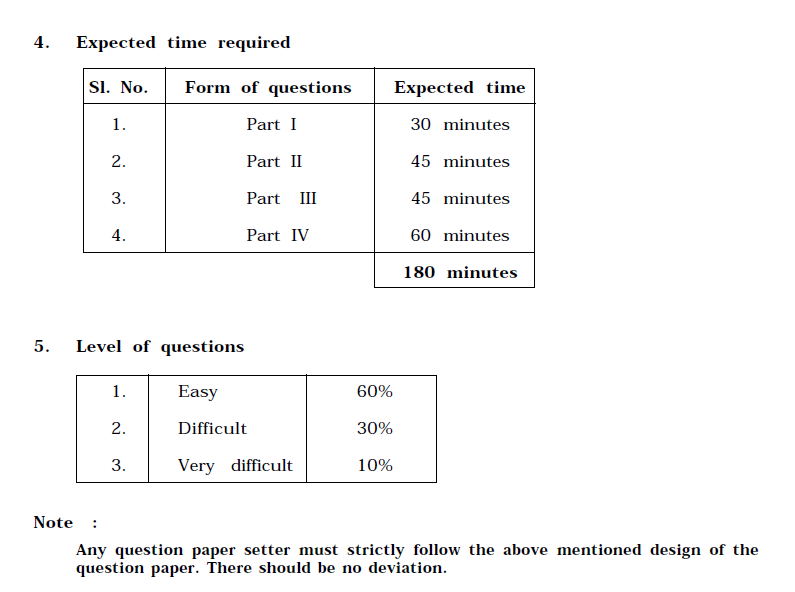# Tamil Nadu Board Class 12 Physics Blue-print and Marking Scheme

Here details have been provided pertaining to the Tamil Nadu Board Class 12 Physics blueprint and exam pattern. Important information such as question paper blueprint, marking scheme and exam pattern with detailed marks weightage are listed below. Students can download this PDF and use it to prepare well for the exams. Knowing the entire Tamil Nadu Board Class 12 Physics Blueprint with Marks Weightage and Exam Pattern will help the students to plan for their exam preparations, accordingly.

We have given an idea about the weightage to learning outcomes, weightage to various types of questions, weightage to various chapters of TN Board Class 12 Physics and so on in this article here.

Check the 12th TN Board Physics Blue-print from here:

Students can also refer to the TN Board Class 12 Physics Sample Papers before preparing for the exam. Other than the blueprint these paper will also give an idea about exam pattern. Meanwhile, take a look at the physics blue print given here.

Design of the TN Board 12th Physics blue-print and marking scheme is given below:

Time: 3 Hours Total Marks: 150

1. Weightage to learning outcomes

 Objective Marks Percentage Knowledge 81 35% Understanding 92 40% Application 46 20% Skill 11 5% Total 230 100%

Note:

(i) Total of 230 marks is inclusive of choice.

(ii) There can be a slight variation of 5%.Note:

(i) Of the total 230 marks, 35 marks are allocated to numerical problems

(In Part I – 5 numerical problems, Part II – 5 numerical problems, Part III – 3

numerical problems)

(ii) In part-III, 3 numerical problems should be given. Out of these three problems,

any one numerical problem should be made compulsory. This compulsory

problem should have an internal choice, provided both the problems must be

from the same chapter (unit)

(iii) Of the 220 marks, at least 60% marks should be allocated for the questions from

solved problems, self evaluation questions and exercise problems. The rest will

be from the content of the textbook

(iv) MCQ – Multiple Choice Question VSA – Very Short Answer

3(b). Weightage to various units (chapters)Note: There might be a slight variation to the extent of 5 marks.The marking scheme of the TN Board class 12 physics paper is given above. For a more detailed insight into the class 12 physics marking scheme, exam pattern or the blue-print, students can download and refer to the PDF given in the article, as well.

Stay tuned with BYJU’S and get more updates about TN board and its resources!